Spring til indhold

## Introduction

This article shows how to use the minted package to format and highlight programming language source code within a LaTeX document, starting with an example:

\documentclass{article}
\usepackage{minted}
\begin{document}
\begin{minted}{python}
import numpy as np

def incmatrix(genl1,genl2):
m = len(genl1)
n = len(genl2)
M = None #to become the incidence matrix
VT = np.zeros((n*m,1), int)  #dummy variable

#compute the bitwise xor matrix
M1 = bitxormatrix(genl1)
M2 = np.triu(bitxormatrix(genl2),1)

for i in range(m-1):
for j in range(i+1, m):
[r,c] = np.where(M2 == M1[i,j])
for k in range(len(r)):
VT[(i)*n + r[k]] = 1;
VT[(i)*n + c[k]] = 1;
VT[(j)*n + r[k]] = 1;
VT[(j)*n + c[k]] = 1;

if M is None:
M = np.copy(VT)
else:
M = np.concatenate((M, VT), 1)

VT = np.zeros((n*m,1), int)

return M
\end{minted}
\end{document}


This example produces the following output: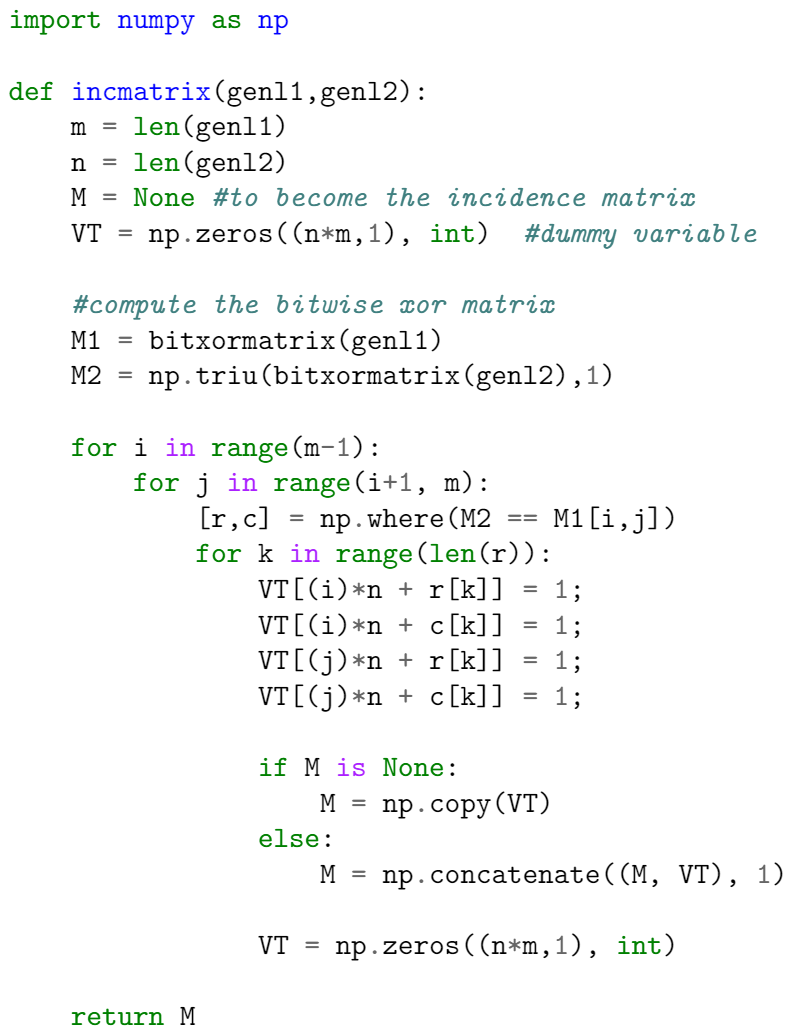There are two important commands here. In the preamble the package is imported by writing

\usepackage{minted}


then the tags \begin{minted}{python} and \end{minted} delimit an environment that print the text verbatim in monospaced fonts and also apply colour to comments, keywords and functions. The parameter python is the programming language the source code is written in. minted supports over 150 programming and markup languages as well as configuration files, see the reference guide for a list of supported languages.

Note: For minted to work with your local LaTeX distribution, an additional program called Pygments must be installed. Overleaf can save you the trouble of installing it and having to run special commands to compile your document—on Overleaf, documents that use minted will work "out of the box".

## Basic usage

As demonstrated in the following example, the minted environment can be configured to modify visual presentation of the typeset code. Here, the minted environment uses several comma-separated parameters of the form key=value:

\documentclass{article}
\usepackage{minted}
\usepackage{xcolor} % to access the named colour LightGray
\definecolor{LightGray}{gray}{0.9}
\begin{document}
\begin{minted}
[
frame=lines,
framesep=2mm,
baselinestretch=1.2,
bgcolor=LightGray,
fontsize=\footnotesize,
linenos
]
{python}
import numpy as np

def incmatrix(genl1,genl2):
m = len(genl1)
n = len(genl2)
M = None #to become the incidence matrix
VT = np.zeros((n*m,1), int)  #dummy variable

#compute the bitwise xor matrix
M1 = bitxormatrix(genl1)
M2 = np.triu(bitxormatrix(genl2),1)

for i in range(m-1):
for j in range(i+1, m):
[r,c] = np.where(M2 == M1[i,j])
for k in range(len(r)):
VT[(i)*n + r[k]] = 1;
VT[(i)*n + c[k]] = 1;
VT[(j)*n + r[k]] = 1;
VT[(j)*n + c[k]] = 1;

if M is None:
M = np.copy(VT)
else:
M = np.concatenate((M, VT), 1)

VT = np.zeros((n*m,1), int)

return M
\end{minted}
\end{document}


This example produces the following output: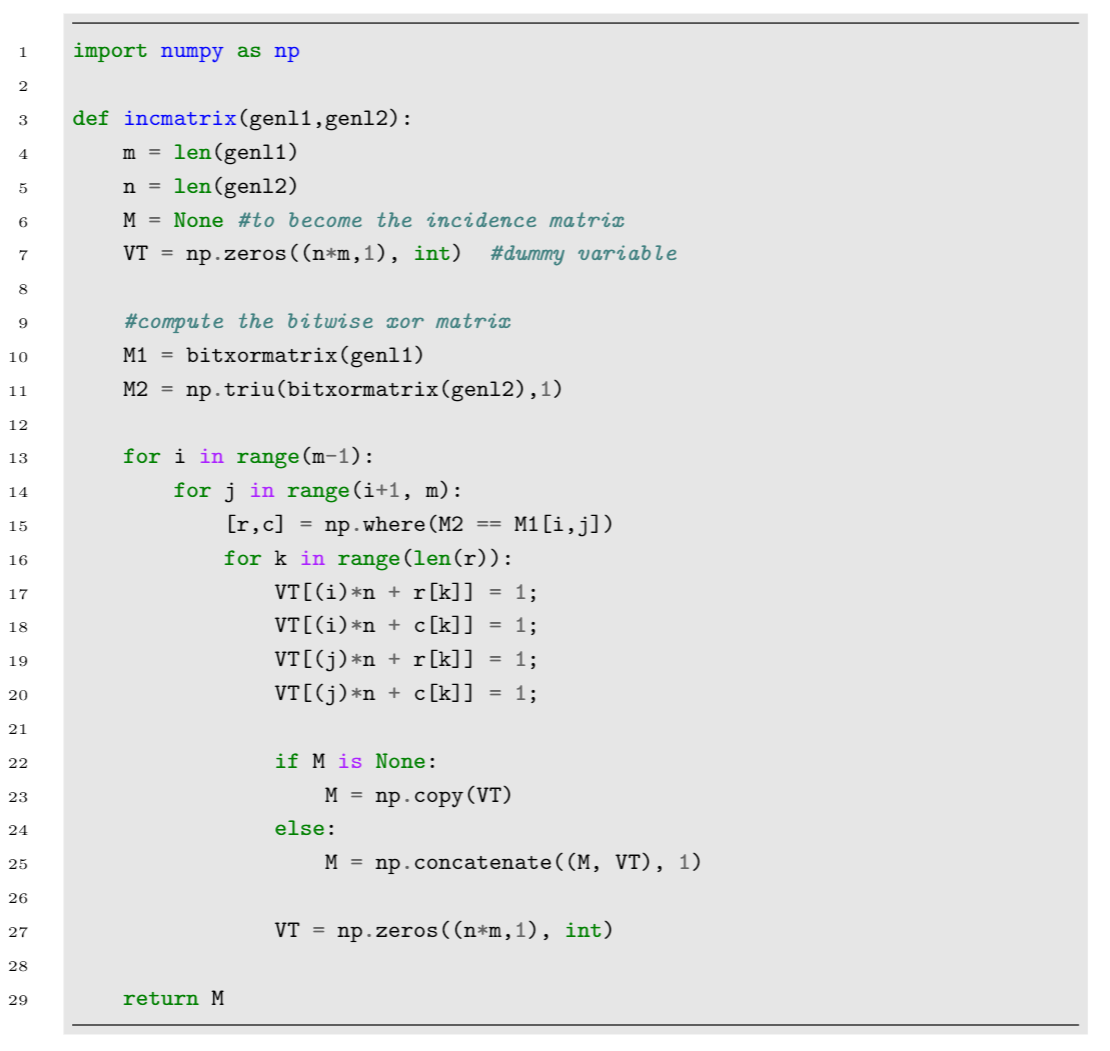The parameters used in this example are:

• frame=lines: draws two lines, one on top and one at the bottom of the code to frame it. Other possible values are leftline, topline, bottomline and single.
• framesep=2mm: the frame separation is set to 2mm. Other length units can be used.
• baselinestretch=1.2: the line spacing of the code set to 1.2.
• bgcolor=LightGray: background colour set to LightGray. You need to import the xcolor package for this to work. See Using colours in LaTeX to learn more about colour manipulation.
• fontsize=\footnotesize: font size set to footnotesize. Any other font size can be set.
• linenos: enables line numbers.

Other options that may be useful are:

• mathescape: enables math mode in code comments.
• rulecolor: changes the colour of the frame.
• showspaces: enables a special character to make spaces visible.

## Including code from a file

Code is usually stored in a source file, therefore a command which automatically imports code from a file is very convenient, as demonstrated in the following example:

\documentclass{article}
\usepackage{minted}
\title{Importing files using minted}
\begin{document}
The next code will be directly imported from a file:

\inputminted{octave}{BitXorMatrix.m}
\end{document}


This example produces the following output: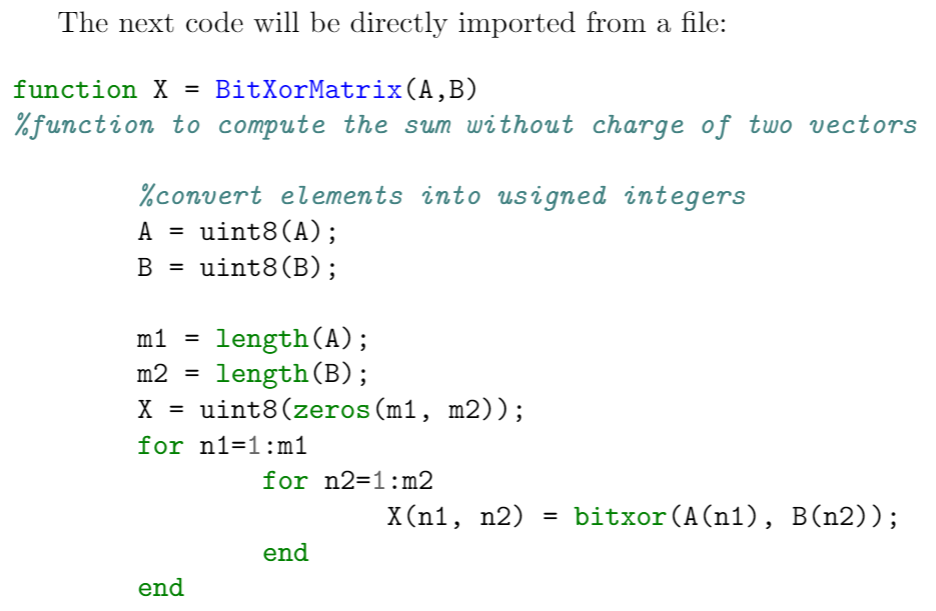The command \inputminted{octave}{BitXorMatrix.m} imports the code from the file BitXorMatrix.m, the parameter octave tells LaTeX the programming language of the code. This command can take two extra parameters to import only part of the file; for instance, to import code from the line 2 to the line 12, the command becomes:

\inputminted[firstline=2, lastline=12]{octave}{BitXorMatrix.m}


## One-line code

If you need to input only a line of code, the command \mint, whose syntax is presented in the next example, will do the trick.

One-line code formatting also works with \texttt{minted}. For example, a small fragment of HTML like this:
\mint{html}|<h2>Something <b>here</b></h2>|
\noindent can be formatted correctly.


This example produces the following output: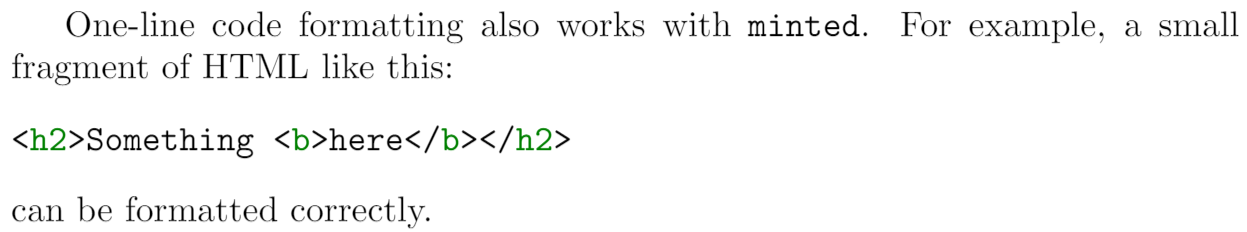The parameter between braces sets the programming language (html markup language in this case) with the actual text to be formatted being delimited by the '|' character.

## Custom lexers

By default, minted supports only languages with lexers that are already installed or registered with pygmentize. If you have written a custom lexer, or want to use a lexer for a language that's not yet been installed on Overleaf, you can still use it in your own Overleaf project using the approach mentioned here.

Suppose you have defined a lexer in the file nl-lexer.py, containing the class NetLogoLexer for the NetLogo language. Upload nl-lexer.py to your Overleaf project, and then specify nl-lexer.py:NetLogoLexer as the "language name" when using minted. For example:

\begin{minted}{nl-lexer.py:NetLogoLexer -x}
\end{minted}


Here's another example for the ImageJ Macro language.

## Colours and stylesheets

The colour schemes used for code highlighting are saved in stylesheets. You can create your own or use one already available in your LaTeX distribution. See the reference guide for a list of stylesheets included in Overleaf.

\documentclass{article}
\usepackage{minted}
\usemintedstyle{borland}
\begin{document}
\begin{minted}{python}
import numpy as np

def incmatrix(genl1,genl2):
m = len(genl1)
n = len(genl2)
M = None #to become the incidence matrix
VT = np.zeros((n*m,1), int)  #dummy variable

#compute the bitwise xor matrix
M1 = bitxormatrix(genl1)
M2 = np.triu(bitxormatrix(genl2),1)

for i in range(m-1):
for j in range(i+1, m):
[r,c] = np.where(M2 == M1[i,j])
for k in range(len(r)):
VT[(i)*n + r[k]] = 1;
VT[(i)*n + c[k]] = 1;
VT[(j)*n + r[k]] = 1;
VT[(j)*n + c[k]] = 1;

if M is None:
M = np.copy(VT)
else:
M = np.concatenate((M, VT), 1)

VT = np.zeros((n*m,1), int)

return M
\end{minted}
\end{document}


Using the borland stylesheet produces the following output: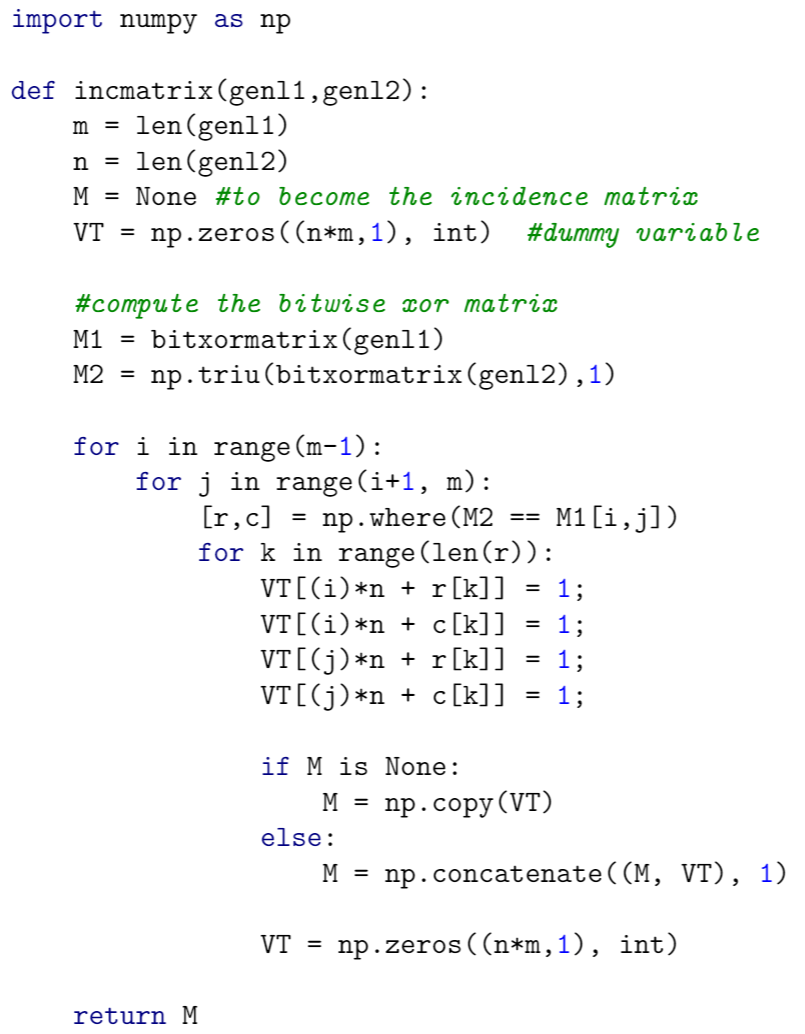The syntax to set a colouring style is easy, the command \usemintedstyle{borland} uses the colour theme borland to format the source code. You can find more colour schemes in the reference guide.

## Captions, labels and the list of listings

Code listings formatted with minted can be included in a float element, just like figures and tables. Captions and labels can be assigned to code listings, and then later be referenced and included in a "List of listings".

\documentclass{article}
\usepackage{minted}
\title{Listing code examples}
\begin{document}
\begin{listing}[!ht]
\inputminted{octave}{BitXorMatrix.m}
\caption{Example from external file}
\label{listing:1}
\end{listing}

\begin{listing}[!ht]
\begin{minted}{c}
#include <stdio.h>
int main() {
printf("Hello, World!"); /*printf() outputs the quoted string*/
return 0;
}
\end{minted}
\caption{Hello World in C}
\label{listing:2}
\end{listing}

\begin{listing}[!ht]
\begin{minted}{lua}
function fact (n)--defines a factorial function
if n == 0 then
return 1
else
return n * fact(n-1)
end
end

print("enter a number:")
print(fact(a))
\end{minted}
\caption{Example from the Lua manual}
\label{listing:3}
\end{listing}
\noindent\texttt{minted} makes a nice job of typesetting listings \ref{listing:1}, \ref{listing:2} and \ref{listing:3}.
\renewcommand\listoflistingscaption{List of source codes}
\listoflistings
\end{document}


The first page of this example contains the following output: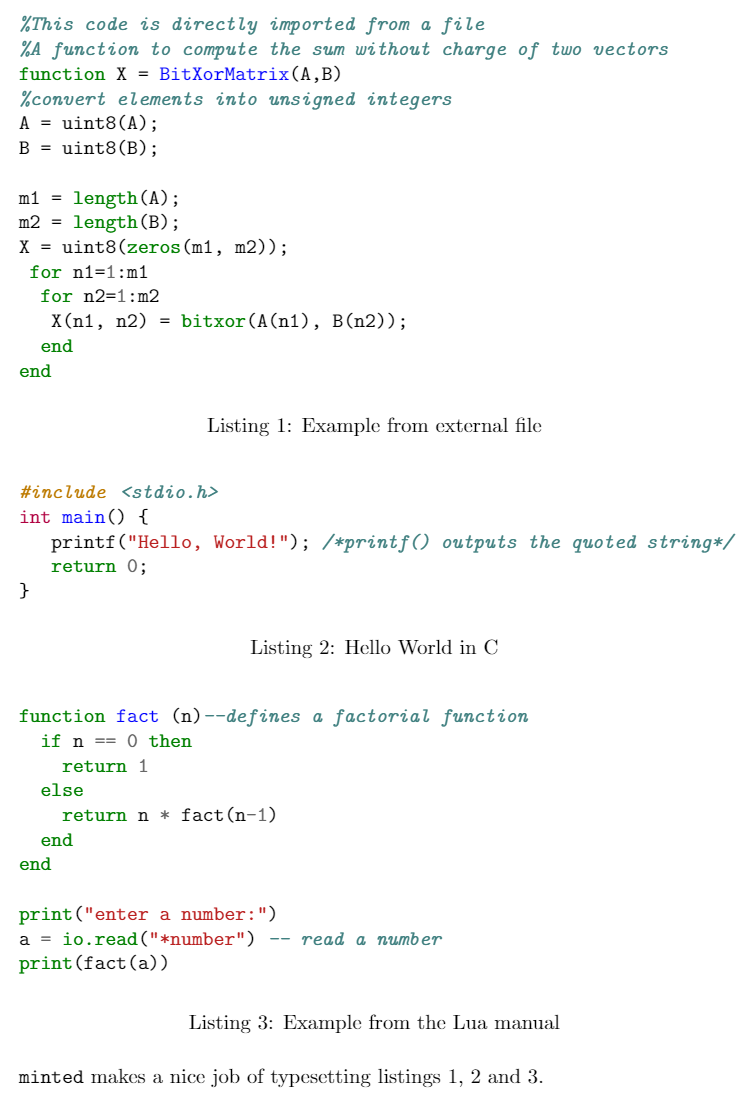To print the list with all "listing" elements use \listoflistings. In the example above, the default title List of listings is changed to List of source codes by writing

\renewcommand\listoflistingscaption{List of source codes}
\listoflistings % Now typeset the list


The second page produced by the example above contains the following listing: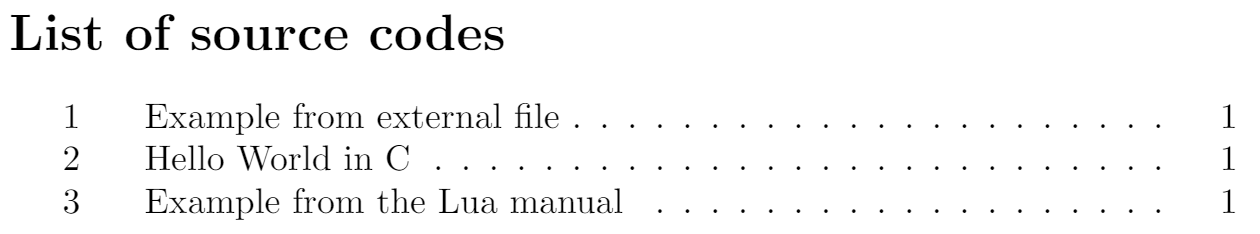## Reference guide

Colour styles for minted

name output name output
manni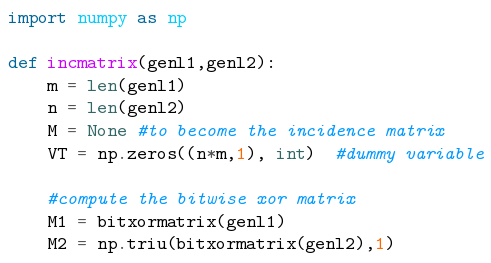fruity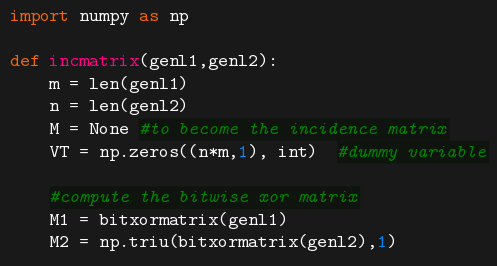rrt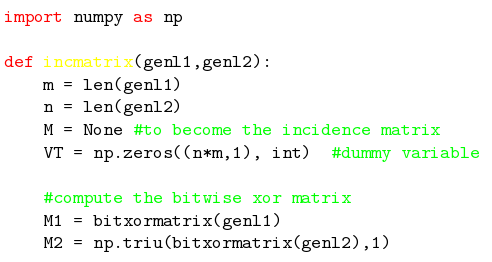autumn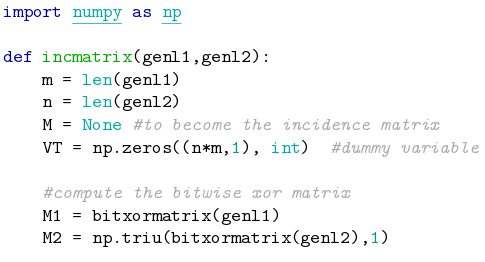perldoc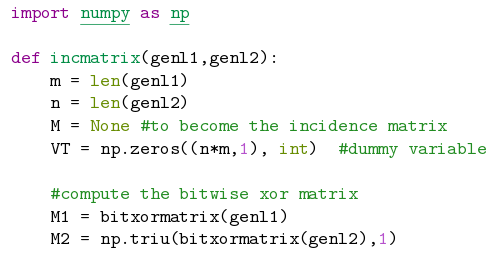bw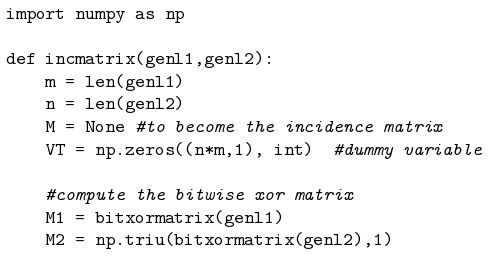borland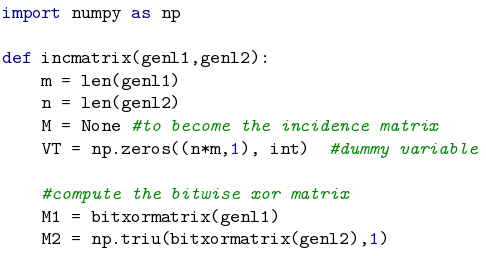emacs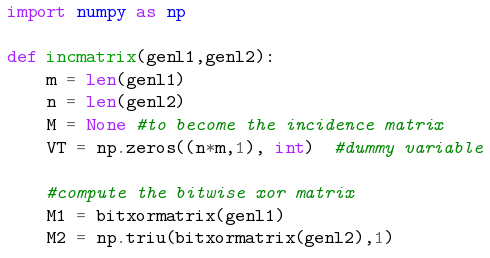colorful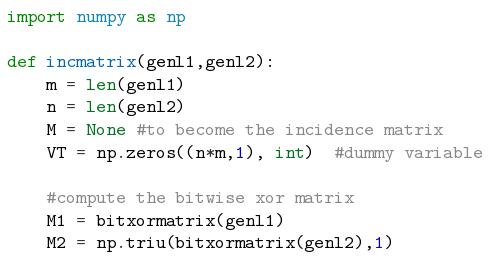vim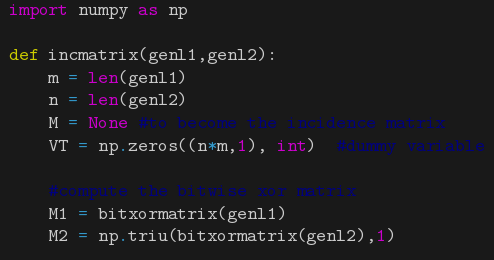murphy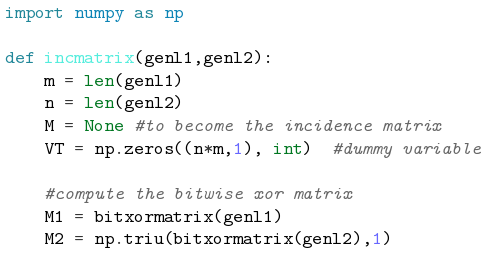pastie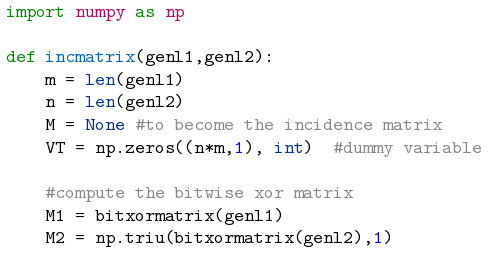vs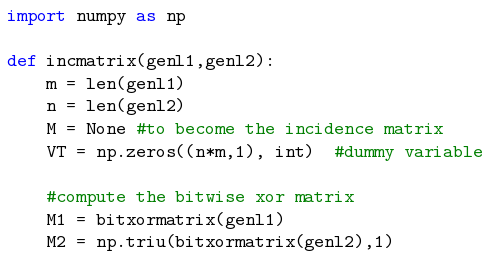friendly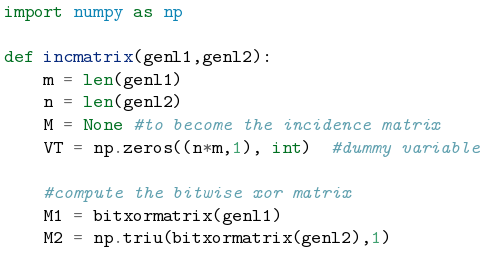trac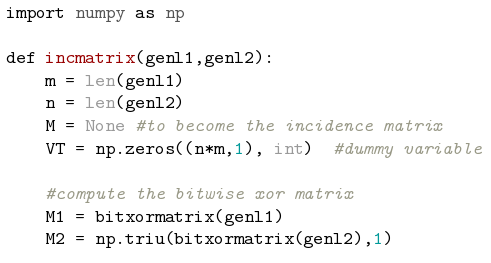native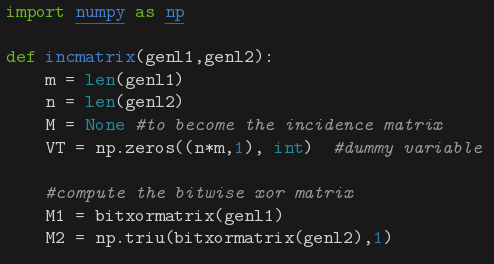tango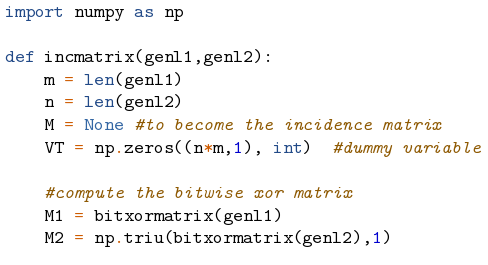monokai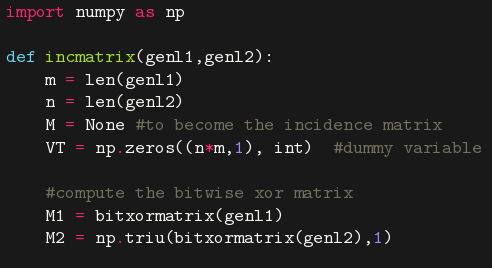Some colour schemes need a dark background to be readable.

Main supported programming languages and configuration files

 cucumber abap ada ahk antlr apacheconf applescript as aspectj autoit asy awk basemake bash bat bbcode befunge bmax boo brainfuck bro bugs c ceylon cfm cfs cheetah clj cmake cobol cl console control coq cpp croc csharp css cuda cyx d dg diff django dpatch duel dylan ec erb evoque fan fancy fortran gas genshi glsl gnuplot go gosu groovy gst haml haskell hxml html http hx idl irc ini java jade js json jsp kconfig koka lasso livescrit llvm logos lua mako mason matlab minid monkey moon mxml myghty mysql nasm newlisp newspeak numpy ocaml octave ooc perl php plpgsql postgresql postscript pot prolog psql puppet python qml ragel raw ruby rhtml sass scheme smalltalk sql ssp tcl tea tex text vala vgl vim xml xquery yaml